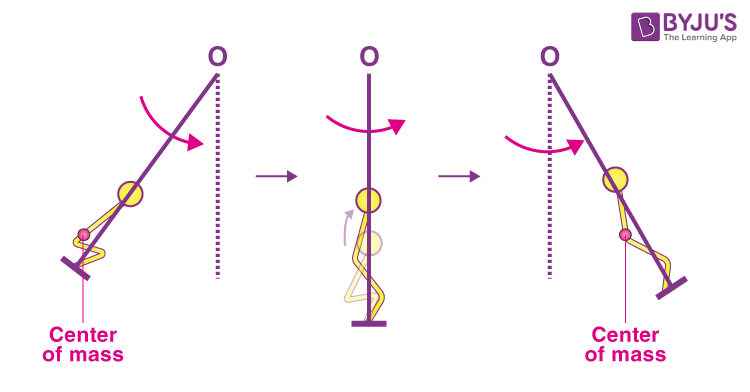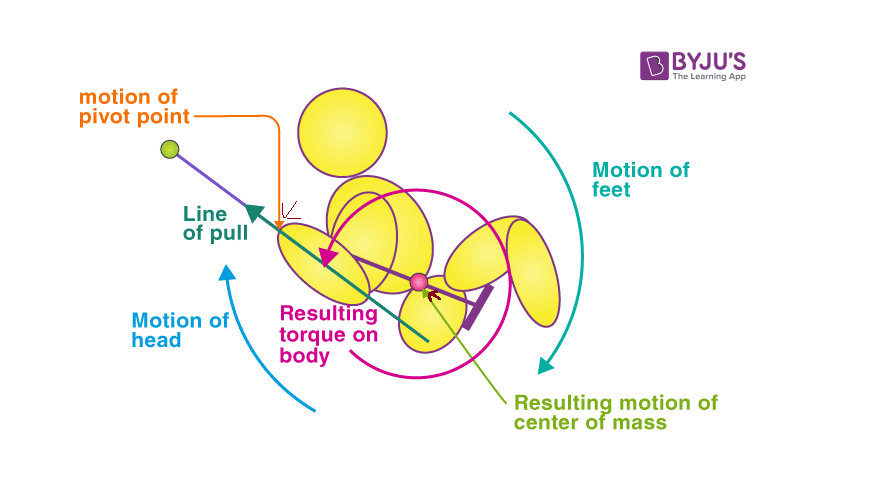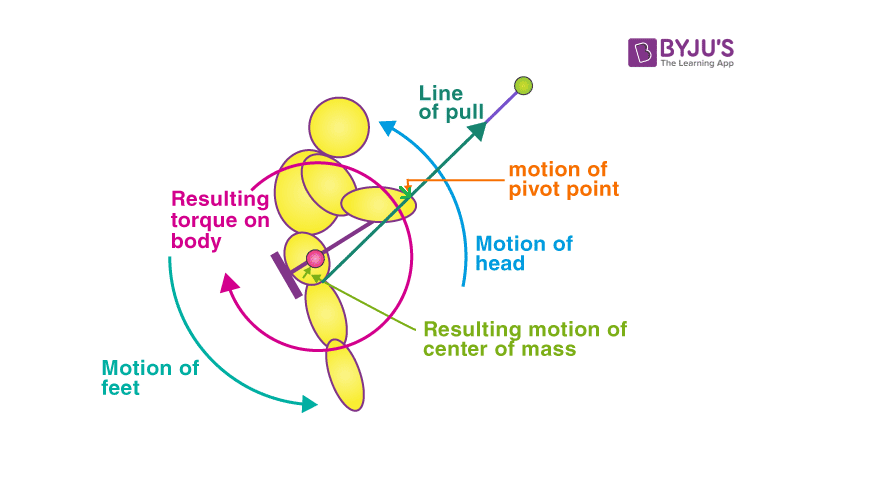Win up to 100% scholarship on Aakash BYJU'S JEE/NEET courses with ABNAT Win up to 100% scholarship on Aakash BYJU'S JEE/NEET courses with ABNAT

# Angular Momentum Conservation on a Swing

## What Is Angular Momentum?

In simple words, angular momentum is the rotational analogue of linear momentum.

$$\begin{array}{l}\text{It is denoted by}\ {\vec{L}}.\end{array}$$
The instantaneous angular momentum relative to the origin can be written in terms of linear momentum.

$$\begin{array}{l}{\vec{L}}=\vec{r}\times \vec{p}\end{array}$$
$$\begin{array}{l}\vec{r}\ \text{is the instantaneous position vector}.\end{array}$$
$$\begin{array}{l}\vec{p}\ \text{is the instantaneous linear momentum}.\end{array}$$

If we consider a rigid body with a fixed axis of rotation, the angular momentum can be written as:

$$\begin{array}{l}\vec{L}= I\vec{\omega }\end{array}$$

Here, ‘I’ is the moment of inertia of the body about the axis of rotation. The rotational analogue of mass is called the moment of inertia. Just like the mass, the moment of inertia determines the rotational kinetic energy.

ω is the angular velocity

$$\begin{array}{l}\text{The rate of change of the angular momentum is called the torque}\ \vec{\tau }.\end{array}$$

Therefore,

$$\begin{array}{l}\sum \vec{\tau }=\frac{d\vec{L}}{dt}\end{array}$$
.

## Conservation of Angular Momentum

For rotational bodies, when there is no external torque provided, the total angular momentum will remain constant. An ice skater spinning is an example of the conservation of angular momentum. The torque or the rotating effect of the force is almost equal to zero because there is negligible friction between the skates and the ice. The friction is exerted very close to the pivot point. Consequently, the skater can spin for a longer period of time. The skater can also increase the spin by pulling their legs and hands inwards. By pulling their arms and legs inwards, the angular momentum will remain constant since the moment of inertia decreases, and the angular velocity increases accordingly.

## Angular Momentum Conservation on a Swing

### (i) In Standing PositionRiding a swing is like riding a pendulum. As the swing rises, its kinetic energy changes to potential energy and as it falls, the potential energy will become kinetic energy again. The only way to pump up the swing is by increasing the total kinetic energy of the pendulum. Where does the extra energy to pump up the swing come from? The extra energy is obtained when the person on the swing changes the position.

The pumped swing is considered a pendulum with a variable-length l. The length l is the distance from the centre of mass of the rider to the fixed swing support point O. Let m be the mass of the rider, τ be the net torque about O due to the gravitational force and the torque about O due to the tension in the string is zero. L is the angular momentum of the body about O.

$$\begin{array}{l}\sum \vec{\tau }=\frac{d\vec{L}}{dt}\end{array}$$

As the rider stands and sits, the effective length l of the swing changes. Assume that the rider squats for the first half of the swing and then instantaneously stands as the swing passes through the vertical position of the swing and remains standing for the upward part of the motion of the swing. The effective length of the swing decreases when the rider stands up as the centre of mass of the rider moves upwards, and squatting will cause an equal increase in the effective length as the centre of mass comes down. The decrease in the effective length of the swing when the rider stands will decrease the moment of inertia of the swing. The angular momentum will be conserved about the fixed point O before and after the rider stands up.

Therefore, L’ = L

I’ω’ = Iω

ω’ = Iω/I’

⇒ ω’ > ω (Since I’ < I)

A decrease in the moment of inertia causes an increase in the angular velocity. In each forcing cycle, the energy of the swing increases as the rider stands from the squat as the swing passes through the vertical position.

### (ii) In Sitting Position

In the sitting position, in order to pump up the swing, the rider changes the orientation of his body with respect to the rope to increase the energy of the swing. At the peak of the arc, the rider throws his head behind and feet forward. This creates an anticlockwise torque to the rider’s head and feet. In response to this torque, the chain of the swing will apply a clockwise torque on the swing and the torso of the rider. So, when the rider throws the head backwards and feet forward, as the rider moves, the angular momentum will increase, and the rider actually gives a thug on the chain, and the centre of mass of the rider will rise slightly. The consequence is that the rider gains potential energy, and it gets converted into kinetic energy when the rider swings down.In the front peak of the arc, the rider bends the head forward and the feet behind. Again there is an increase in energy because the centre of mass gets lifted slightly. As the rider swings back towards the vertical position, the extra potential energy gained will turn into kinetic energy and the extra bit of torque from the chain will result in an increased angular momentum along the vertical position. Therefore, in each to and fro motion, the energy of the swing increases.

## Related Videos

### Angular Momentum Conservation on a Swing### Conservation of Angular Momentum### Problem on Conservation of Angular Momentum## Frequently Asked Questions on Angular Momentum Conservation on a Swing

Q1

### Is angular momentum conserved?

When there is no net external torque, angular momentum is conserved, just as linear momentum is conserved when there is no net external force.

Q2

### Give an example of the conservation of angular momentum.

An ice skater spins and changes the speed of her rotation by pushing her arms outwards or holding them inwards is an example of conservation of angular momentum.

Q3

### Explain the term angular momentum.

The velocity of rotation of something around an axis is known as spin or angular momentum.